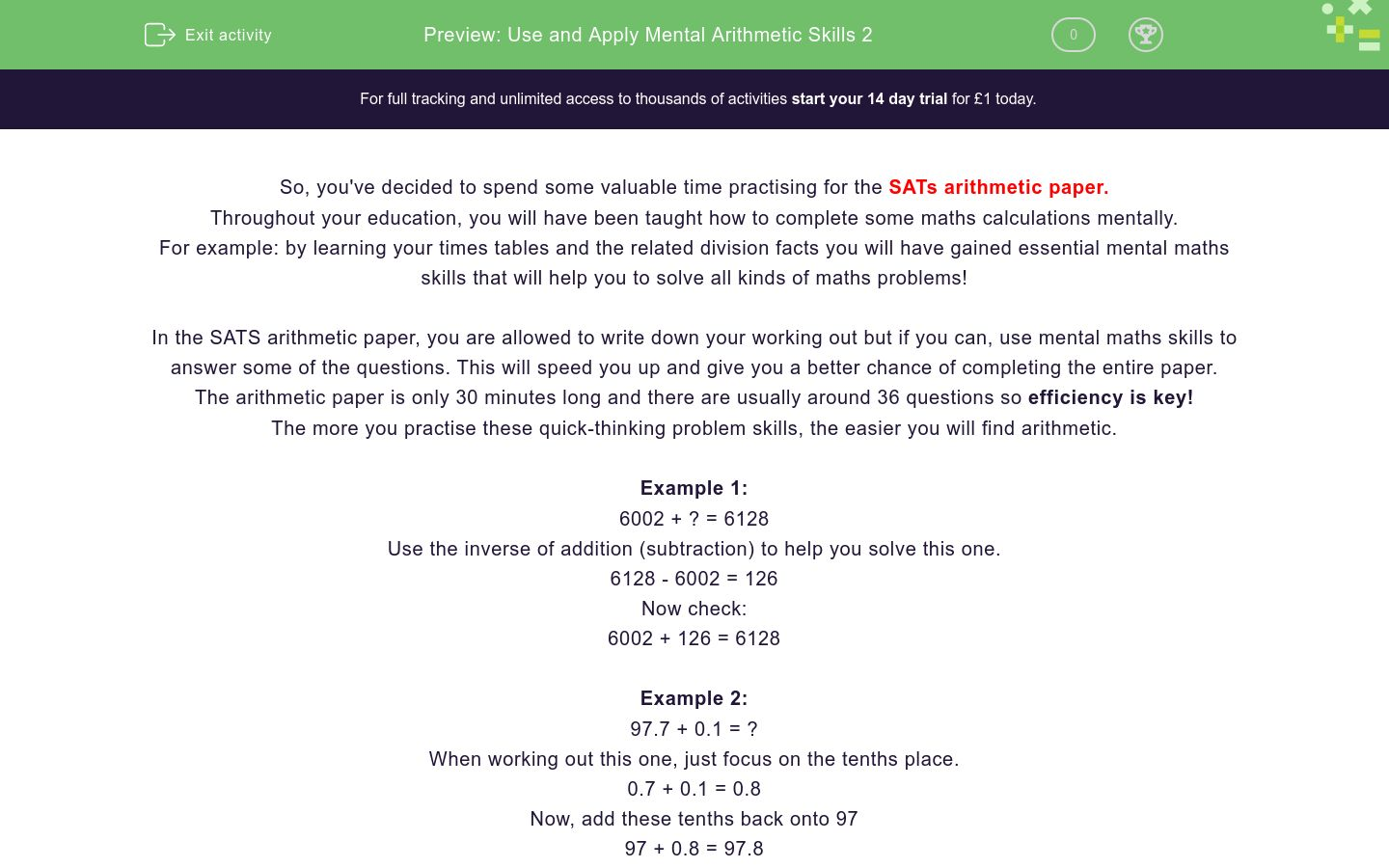# Use and Apply Mental Arithmetic Skills 2

In this worksheet, students will practise foundational mental maths skills in preparation for their SATs arithmetic paper. This worksheet will guide students through specific strategies enabling them to confidently solve problems mentally.Key stage:  KS 2

Curriculum topic:   Exam-style Questions: SATs Maths

Curriculum subtopic:   Exam-Style Questions: Mental Maths

Difficulty level:### QUESTION 1 of 10

So, you've decided to spend some valuable time practising for the SATs arithmetic paper.

Throughout your education, you will have been taught how to complete some maths calculations mentally.

For example: by learning your times tables and the related division facts you will have gained essential mental maths skills that will help you to solve all kinds of maths problems!

In the SATS arithmetic paper, you are allowed to write down your working out but if you can, use mental maths skills to answer some of the questions. This will speed you up and give you a better chance of completing the entire paper.

The arithmetic paper is only 30 minutes long and there are usually around 36 questions so efficiency is key!

The more you practise these quick-thinking problem skills, the easier you will find arithmetic.

Example 1:

6002 + ? = 6128

6128 - 6002 = 126

Now check:

6002 + 126 = 6128

Example 2:

97.7 + 0.1 = ?

When working out this one, just focus on the tenths place.

0.7 + 0.1 = 0.8

Now, add these tenths back onto 97

97 + 0.8 = 97.8

Over to you now to try a mixture of quick-fire arithmetic questions!

3/6 + 2/6 =

2/6

3/6

1/6

5/6

5181 - 100 = ?

87.8 - 3.2 = ?

90.6

85.6

83.6

84.6

Can you match the answers with their corresponding calculation?

## Column B

40 ÷ 8 =
500
300 ÷ 6 =
50
1000 ÷ 2 =
36,000
12,000 x 3 =
5

7² - 12 = ?

447 x 9 = ?

42,000 ÷ 7 = ?

6000

7000

9000

8000

Can you solve the different calculations?

6000

7000

9000

8000

What is 20% of 940?

6000

7000

9000

8000

(8 x 6) ÷ 2 = ?

• Question 1

3/6 + 2/6 =

5/6
EDDIE SAYS
Brilliant start if you chose 5/6. Remember, when adding fractions, ensure the denominator (bottom numbers) are the same. Then, add the top numbers and put the total over the same denominator.
• Question 2

5181 - 100 = ?

5081
5 081
5,081
EDDIE SAYS
Did you get the hang of it? This time we had to subtract 100. Focus on the hundreds column, to begin with: 100 - 100 = 0. Then, put the remaining, untouched digits back into their correct place, leaving us with 5081.
• Question 3

87.8 - 3.2 = ?

84.6
EDDIE SAYS
Did you reach the answer of 84.6? Use partitioning to help you to complete this subtraction. Step 1: Focus on the ones column: 7 - 3 = 4 Step 2: Focus on the tenths column: 0.8 - 0.2 = 0.6 Step 3: Place the digits back into their correct place: 84.6 Great place-value knowledge helps you to answer these kinds of questions quickly and accurately.
• Question 4

Can you match the answers with their corresponding calculation?

## Column B

40 ÷ 8 =
5
300 ÷ 6 =
50
1000 ÷ 2 =
500
12,000 x 3 =
36,000
EDDIE SAYS
Hopefully, you were able to use your knowledge of times tables and related division facts to correctly match the answer for each calculation.
• Question 5

7² - 12 = ?

37
EDDIE SAYS
Did you remember what 7² means? 7² requires us to multiply 7 by itself. 7 x 7 = 49 49 - 12 = 37.
• Question 6

447 x 9 = ?

4023
4,023
4 023
EDDIE SAYS
A quick shortcut for this question is to calculate 447 x 10 = 4470. Then we subtract 447 from 4470: 9 x 447 = 4023. The written methods you could use to solve this question would be column multiplication or grid method multiplication. Choose the method that will help you to complete the question most efficiently in the test- whichever works best for you!
• Question 7

42,000 ÷ 7 = ?

6000
EDDIE SAYS
Did you find the answer 6,000? An efficient method is to fall back upon your trusted times tables! 42 ÷ 7 = 6 420 ÷ 7 = 60 4200 ÷ 7 = 600 42,000 ÷ 7 = 6000.
• Question 8

Can you solve the different calculations?

EDDIE SAYS
Don't worry if you found this challenging. Take your time and think carefully about how many spaces to the left or right the digits should move. When you multiply, the number becomes larger and all digits move to the left. When you divide, the number becomes smaller and all digits move to the right.
• Question 9

What is 20% of 940?

EDDIE SAYS
Phew, that was a challenge! When you're asked to find a percentage of a number, you should always find 10% first as it's simple! 10% of 940 = 940 ÷ 10 = 94 Double 94 to find 20% = 94 x 2 = 188.
• Question 10

(8 x 6) ÷ 2 = ?

24
EDDIE SAYS
(8 x 6) ÷ 2 = ? Remember BODMAS! Complete any calculation in brackets first. Step 1: 8 x 6 = 48 Step 2: Complete division 48 ÷ 2 = 24 Great focus, that's another activity completed and you're now more prepared for the SATs arithmetic test.